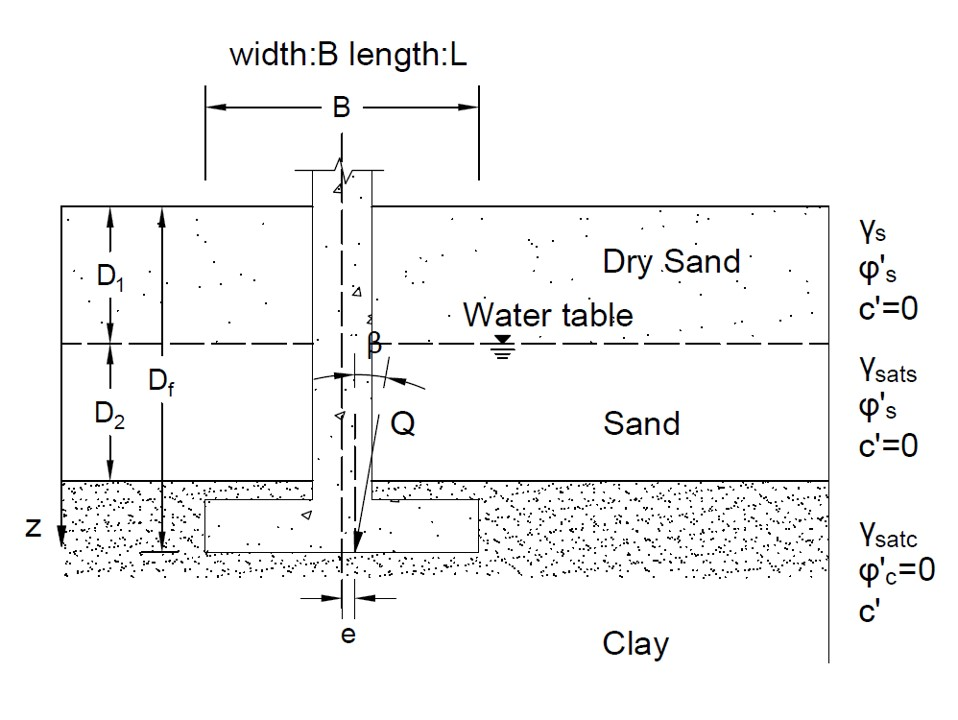# Shallow Foundation Tool (qu)## Geometry of shallow foundation (bearing capacity)## Tips

• This tool is for evaluating the bearing capacity of shallow foundation, as shown in the figure above.
• All the input parameters should be positive.
• The unit weight of water is 9.8 kN/m3.
• The depth of the shallow foundation bottom Df can be smaller than (D1+D2).
• For continuous foundation (strip footing), please input a large value (>20B) or "continuous" for L.
• If the foundation is in clay, φ=0 and c=cu. If the foundation is in sand, c=0.
• If no inclined loading is applied, just input β = 0. If no eccentric loading is applied, just input e = 0.
• The angle of inclination and the eccentricity of loading should not be too big (β < 45 & e < B/6), or the design should be optimized.
• For "nan", "0" or "inf" displayed in Results, please check your input parameters.
• If Qall is given to estimate Fs, users can try to input different Fs to see the "Qall" in Results. The real Fs corresponds to the caluclated "Qall" same as the given Qall.
• Same logic of trial-error process can be used to estimate the dimension of the foundation (width & depth) for a given Qall and a required Fs.

B (m)
L (m)
D1 (m)
D2 (m)
Df (m)
β (°)
e (m)
γs (kN/m3)
γsat_s (kN/m3)
γsat_c (kN/m3)
φ′ (°)
c (kPa)
Fs